# ROC曲线的绘制与AUC的计算2019年7月9日07:38:3541 7171字阅读23分54秒

## 简介

### 参考资料

sklearn计算绘图代码例子(我自己主要就是参考得这个链接) : Receiver Operating Characteristic (ROC)

ROC原理讲解 : Introduction to ROC Curves

## ROC介绍

ROC curves typically feature true positive rate on the Y axis, and false positive rate on the X axis. This means that the top left corner of the plot is the "ideal" point - a false positive rate of zero, and a true positive rate of one. This is not very realistic, but it does mean that a larger area under the curve (AUC) is usually better.

The "steepness" of ROC curves is also important, since it is ideal to maximize the true positive rate while minimizing the false positive rate.

### ROC名字来源

You may be wondering where the name "Reciever Operating Characteristic" came from. ROC analysis is part of a field called "Signal Dectection Theory" developed during World War II for the analysis of radar images.

Radar operators had to decide whether a blip on the screen represented an enemy target, a friendly ship, or just noise. Signal detection theory measures the ability of radar receiver operators to make these important distinctions. Their ability to do so was called the Receiver Operating Characteristics. It was not until the 1970's that signal detection theory was recognized as useful for interpreting medical test results.

## ROC曲线的绘制与AUC的计算--例子

### 整体解释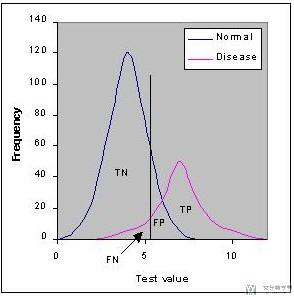### 预备知识

TPR (True Positive Rate) / Recall /Sensitivity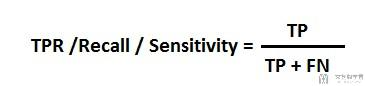Specificity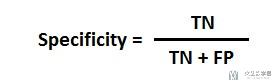FPR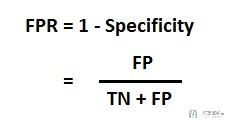### ROC曲线的绘制(具体的算例)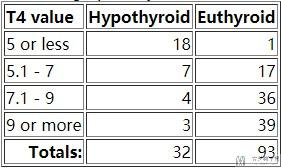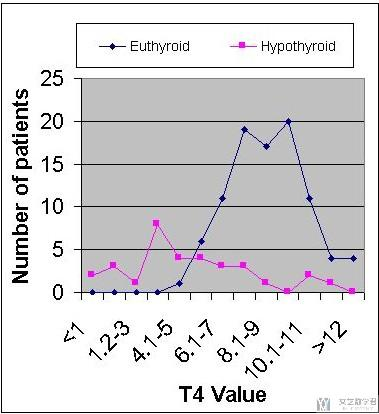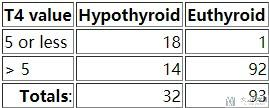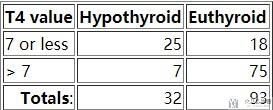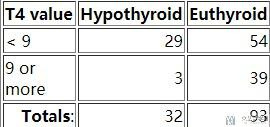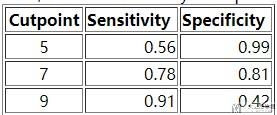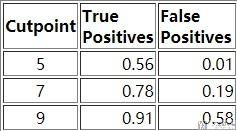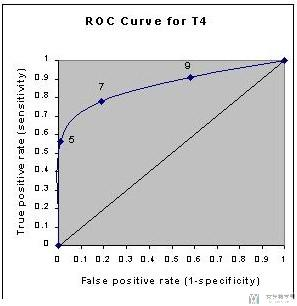• 当阈值T4 Value我们调整为最大的时候，这个时候就是全部预测为甲状腺功能异常(Hypothyroid)，此时的TPR=FPR=1；
• 当阈值T4 Value我们调整为最小的时候，这个时候就是全部预测为甲状腺功能正常(Euthyroid)，此时的TPR=FPR=0；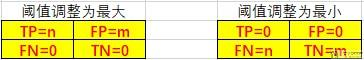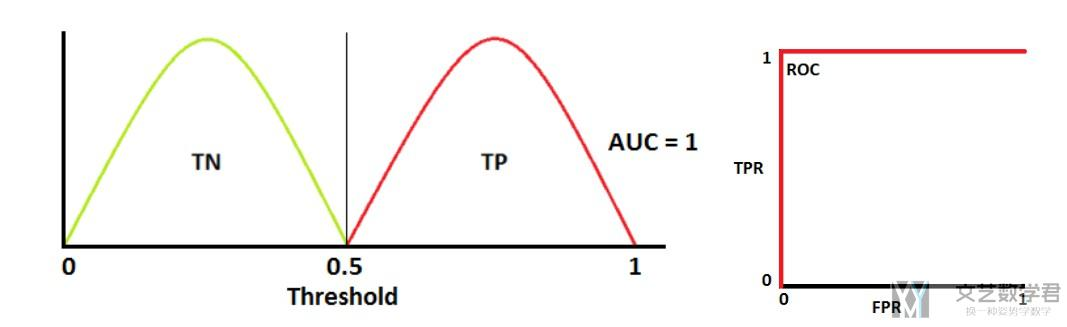### AUC值的计算(具体算例)

Accuracy is measured by the area under the ROC curve. AUC的值就是ROC曲线的下半部分。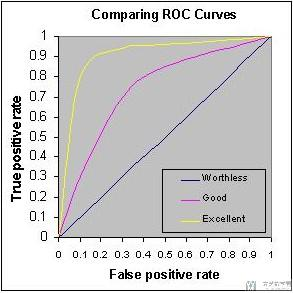## ROC曲线绘制与AUC值计算(代码介绍)

### 一个例子(官方样例)

#### 模型的训练

1. import numpy as np
2. import matplotlib.pyplot as plt
3. from itertools import cycle
4. from sklearn import svm, datasets
5. from sklearn.metrics import roc_curve, auc
6. from sklearn.model_selection import train_test_split
7. from sklearn.preprocessing import label_binarize
8. from sklearn.multiclass import OneVsRestClassifier
9. from scipy import interp
10. # Import some data to play with
12. X = iris.data
13. y = iris.target
14. # Binarize the output
15. y = label_binarize(y, classes=[0, 1, 2])
16. n_classes = y.shape
17. # Add noisy features to make the problem harder
18. random_state = np.random.RandomState(0)
19. n_samples, n_features = X.shape
20. X = np.c_[X, random_state.randn(n_samples, 200 * n_features)]
21. # shuffle and split training and test sets
22. X_train, X_test, y_train, y_test = train_test_split(X, y, test_size=.5,
23.                                                     random_state=0)
24. # Learn to predict each class against the other
25. classifier = OneVsRestClassifier(svm.SVC(kernel='linear', probability=True,
26.                                  random_state=random_state))
27. y_score = classifier.fit(X_train, y_train).decision_function(X_test)

#### 计算每一个AUC值(包括micro和macro)

1. # Compute ROC curve and ROC area for each class
2. fpr = dict()
3. tpr = dict()
4. roc_auc = dict()
5. for i in range(n_classes):
6.     fpr[i], tpr[i], _ = roc_curve(y_test[:, i], y_score[:, i])
7.     roc_auc[i] = auc(fpr[i], tpr[i])
8. # 这个AUC值
9. print(roc_auc)
10. # Compute micro-average ROC curve and ROC area
11. fpr["micro"], tpr["micro"], _ = roc_curve(y_test.ravel(), y_score.ravel()) # 这个是ROC曲线的坐标
12. roc_auc["micro"] = auc(fpr["micro"], tpr["micro"]) # 这个是计算AUC的值
13. print(roc_auc)

1. # Compute macro-average ROC curve and ROC area
2. # First aggregate all false positive rates
3. all_fpr = np.unique(np.concatenate([fpr[i] for i in range(n_classes)]))
4. # Then interpolate all ROC curves at this points
5. mean_tpr = np.zeros_like(all_fpr)
6. for i in range(n_classes):
7.     mean_tpr += interp(all_fpr, fpr[i], tpr[i])
8. # Finally average it and compute AUC
9. mean_tpr /= n_classes
10. fpr["macro"] = all_fpr
11. tpr["macro"] = mean_tpr
12. roc_auc["macro"] = auc(fpr["macro"], tpr["macro"])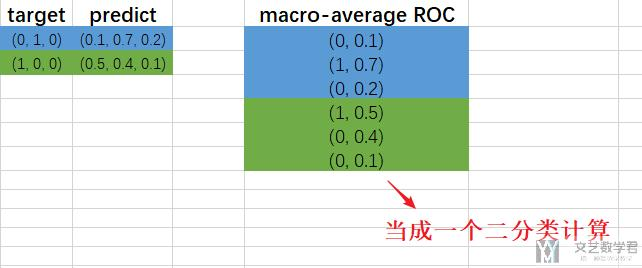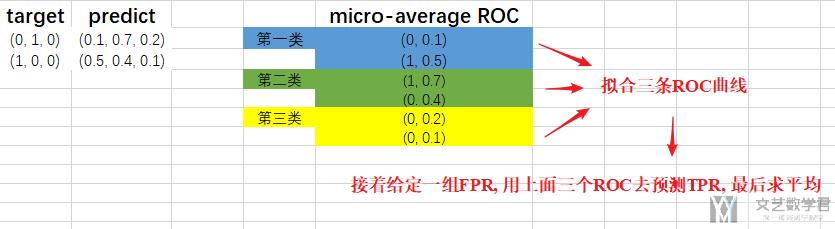#### 绘制ROC曲线和计算AUC值

1. plt.figure()
2. lw = 2 # linewidth(线条的粗细)
3. plt.plot(fpr, tpr, color='darkorange',
4.          lw=lw, label='ROC curve (area = %0.2f)' % roc_auc)
5. plt.plot([0, 1], [0, 1], color='navy', lw=lw, linestyle='--') # 这是绘制中间的直线
6. plt.xlim([0.0, 1.0])
7. plt.ylim([0.0, 1.05])
8. plt.xlabel('False Positive Rate')
9. plt.ylabel('True Positive Rate')
11. plt.legend(loc="lower right")
12. plt.show()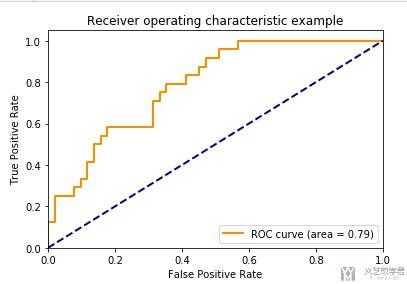1. # Plot all ROC curves
2. plt.figure()
3. plt.plot(fpr["micro"], tpr["micro"],
4.          label='micro-average ROC curve (area = {0:0.2f})'
5.                ''.format(roc_auc["micro"]),
6.          color='deeppink', linestyle=':', linewidth=4)
7. plt.plot(fpr["macro"], tpr["macro"],
8.          label='macro-average ROC curve (area = {0:0.2f})'
9.                ''.format(roc_auc["macro"]),
10.          color='navy', linestyle=':', linewidth=4)
11. colors = cycle(['aqua', 'darkorange', 'cornflowerblue'])
12. for i, color in zip(range(n_classes), colors):
13.     plt.plot(fpr[i], tpr[i], color=color, lw=lw,
14.              label='ROC curve of class {0} (area = {1:0.2f})'
15.              ''.format(i, roc_auc[i]))
16. plt.plot([0, 1], [0, 1], 'k--', lw=lw)
17. plt.xlim([0.0, 1.0])
18. plt.ylim([0.0, 1.05])
19. plt.xlabel('False Positive Rate')
20. plt.ylabel('True Positive Rate')
21. plt.title('Some extension of Receiver operating characteristic to multi-class')
22. plt.legend(loc="lower right")
23. plt.show()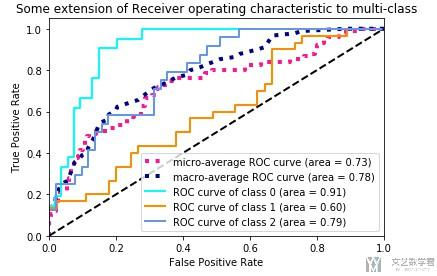• 微信公众号
• 关注微信公众号
•• QQ群
• 我们的QQ群号
•• 本文由 发表于 2019年7月9日07:38:35
• 转载请务必保留本文链接：https://mathpretty.com/10743.html

•郭鑫宇

作者您好，我想问一下，单独画出来的ROC曲线有什么重要意义吗？ 还想再问一下，您已经说了微观平均ROC的意义，但宏观平均ROC的意义还没说呢。嘻嘻，求教啦。
我部分引用了您的文章。感谢您。
https://www.cnblogs.com/guoxinyu/p/12687484.html

•王 茂南

@ 郭鑫宇 你好, 感谢你的评论. 我已经在原文中进行了更进一步的说明, 你可以重新读一下那部分, 我增加了文字和图片的介绍.

•郭鑫宇

嘻嘻作者您好。我更新了自己的文章。不过感觉您的微观宏观的叙述，和新作的Excel图好像颠倒了嘿。

点击我的昵称即可进入原文

•王 茂南

@ 郭鑫宇 是的, Excel图写反了, 我在文章里做一下文字说明, 图片就不改了. 谢谢啦.